## Law of Cosines

The previous section covered the solving of right triangles. In this section, and the next, you see formulas that can be solve any triangle. If α, β, and γ are the angles of any (right, acute, or obtuse) triangle, and a, b, and c are the lengths of the three sides opposite α, β, and γ, respectively, then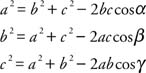These three formulas are called the Law of Cosines. Each follows from the distance formula and is illustrated in Figure 1.Figure 1
Reference triangle for Law of Cosines.

From the figure,Thus the coordinates of A areRemember, all three forms of the Law of Cosines are true even if γ is acute. Using the distance formula,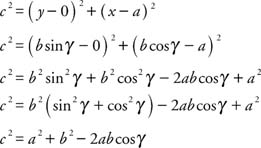In the preceding formula, if γ is 90°, then the cos 90° = 0, yielding the Pythagorean theorem for right triangles. If the orientation of the triangle is changed to have A or B at the origin, then the other two versions of the Law of Cosines can be obtained.

Two specific cases are of particular importance. First, use the Law of Cosines to solve a triangle if the length of the three sides is known.

Example 1: If α, β, and γ are the angles of a triangle, and a, b, and c are the lengths of the three sides opposite α, β, and γ, respectively, and a = 12, b = 7, and c = 6, then find the measure of β.

Use the form of the Law of Cosines that uses the angle in question.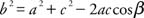Rewrite solving for cos β.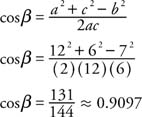Because cos β > 0 and β < 180°, β < 90° thus,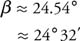The measure of α can be found in a similar way.Rewrite solving for cos α.Because cos α < 0 and α 180° α > 90° Thus,Because the three angles of the triangle must add up to 180°,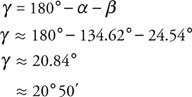Next, solve a triangle knowing the lengths of two sides and the measure of the included angle. First, find the length of the third side by using the Law of Cosines. Then proceed as in Example 7 to find the other two angles.

Example 2: Using Figure 2 , find the length of side b.Figure 2
Drawing for Example 2.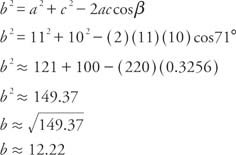Example 3: Find the area of the triangle in Example 8.

First reposition the triangle as shown in Figure so that the known angle is in standard position.Figure 3
Drawing for Example 3.

The base of the triangle is 11. You can find the height of the triangle by using the fact thatTherefore,